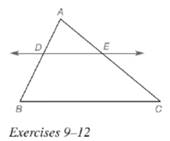Chapter 5.6, Problem 12EElementary Geometry For College St...

7th Edition
Alexander + 2 others
ISBN: 9781337614085

Solutions

Chapter
SectionElementary Geometry For College St...

7th Edition
Alexander + 2 others
ISBN: 9781337614085
Textbook Problem

Given: D E ↔ ∥ B C , ¯ A D = 5 , D B = a + 3 , A E = a + 1 , E C = 3 ( a − 1 ) Find: a  and  E CTo determine

To find:

a,EC.

Explanation

Given:

Theorem used:

If a line is parallel to one side of a triangle and intersects other two sides, then it divides these sides proportionally.

Calculation:

Given: DEBC¯

Since if a line is parallel to one side of a triangle and intersects other two sides, then it divides these sides proportionally.

Also given that AD=5,DB=a+3,AE=a+1,EC=3(a1).

Thus 5a+3=a+13(a1).

Now let us find the value of a.

5a+3=a+13(a1)15(a1)=(a+1)(a+3)15

Still sussing out bartleby?

Check out a sample textbook solution.

See a sample solution

The Solution to Your Study Problems

Bartleby provides explanations to thousands of textbook problems written by our experts, many with advanced degrees!

Get Started

In problems 1-16, solve each equation. 11. Solve

Mathematical Applications for the Management, Life, and Social Sciences

Let f(x) = logb (3x2 2). For what value of b is f(1) = 3?

Single Variable Calculus: Early Transcendentals, Volume I

True or False: converges.

Study Guide for Stewart's Multivariable Calculus, 8th

Suppose that P(E)=.60, P(F)=.32, and P(EF)=.22. Are E and F independent?

Finite Mathematics for the Managerial, Life, and Social Sciences